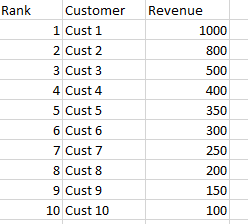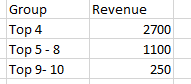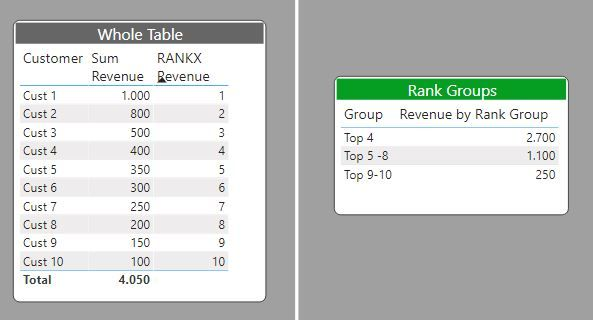cancel
Showing results for
Did you mean:Helper I

## How to group customers dynamically based on slicer values

Hi,

i am having a requirement to dynamically group customers.

I will have 3 sclicers Top,Group1,Group2. Based on Top slicer value the TopN customers should display in one table.

I could able to do that. Sample image for reference.but i need to show another table like below screenshotRow1 should group based on Group1 slicer value, row2 should group between group1 and group2 slicer values, the rest should group as row 3.

I have tried in multiple ways but no luck.

1 ACCEPTED SOLUTIONSuper User

Create a table using the "Enter data" option in the Home ribbon and type in the groups (let's call it 'Grouping Table'):

Group       Order

Top 4           1

Top 5-8.       2

Top 9-10      3

(Use the order field to sort the table: select the column Group and use the "Sort column by" option in table view)

You can use this table as a slicer if need be.

Next create a measure including the grouping values:

Grouping Values=

VAR Top4 = CALCULATE([Revenue], FILTER(Table,  [Rank] < 5))

VAR Top5to8 = CALCULATE([Revenue], FILTER(Table, [Rank] > 4 && [Rank] < 9))

VAR Top9to10 = CALCULATE([Revenue], FILTER(Table, [Rank]>8 && [Rank] <11))

RETURN

Switch(TRUE(),

Grouping Table [Group] = "Top 4", Top4,

Grouping Table[Group] = "Top 5-8", Top5to8,

Top9to10)

now create a table visual with the Grouping Table[Group] field and the [Grouping Values] measure

In doing so, you are also helping me. Thank you!

Proud to be a Super User!

4 REPLIES 4Community Support

If the above posts help, please kindly mark it as a answer to help others find it more quickly. thanks!

If not, please kindly elaborate more.

Community Support Team _ Dina Ye
If this post helps, then please consider Accept it as the solution to help the other members find it more
quickly.Super User

Create a table using the "Enter data" option in the Home ribbon and type in the groups (let's call it 'Grouping Table'):

Group       Order

Top 4           1

Top 5-8.       2

Top 9-10      3

(Use the order field to sort the table: select the column Group and use the "Sort column by" option in table view)

You can use this table as a slicer if need be.

Next create a measure including the grouping values:

Grouping Values=

VAR Top4 = CALCULATE([Revenue], FILTER(Table,  [Rank] < 5))

VAR Top5to8 = CALCULATE([Revenue], FILTER(Table, [Rank] > 4 && [Rank] < 9))

VAR Top9to10 = CALCULATE([Revenue], FILTER(Table, [Rank]>8 && [Rank] <11))

RETURN

Switch(TRUE(),

Grouping Table [Group] = "Top 4", Top4,

Grouping Table[Group] = "Top 5-8", Top5to8,

Top9to10)

now create a table visual with the Grouping Table[Group] field and the [Grouping Values] measure

In doing so, you are also helping me. Thank you!

Proud to be a Super User!Helper I

Hi,

I have tried this kind of approach only, but Top5to8,Top9to10 values coming wrong.

The dataset will limit based on Top slicer value. So i will have only top 10 customers, on this top 10 i need to do groups.Super User

Does this work for you?The Rank Grouping Table is:The RANKX measure:

``````RANKX Revenue =
VAR calc = RANKX(ALL(Data), [Sum Revenue], , DESC)
RETURN
IF(ISINSCOPE(Data[Customer ]), calc)``````

And the final measure to be used in the table visual:

``````Revenue by Rank Group =
VAR Top4 = CALCULATE([Sum Revenue], FILTER(Data, [RANKX Revenue] <5))
VAR Top5to8 = CALCULATE([Sum Revenue], FILTER(Data, [RANKX Revenue] > 4 && [RANKX Revenue] <9))
VAR Top9to10 = CALCULATE([Sum Revenue], FILTER(Data, [RANKX Revenue] > 8 && [RANKX Revenue] <11))
RETURN
SWITCH(TRUE(),
MAX('Rank Grouping'[Oder]) = 1, Top4,
MAX('Rank Grouping'[Oder]) = 2, Top5to8,
Top9to10)``````

In doing so, you are also helping me. Thank you!

Proud to be a Super User!